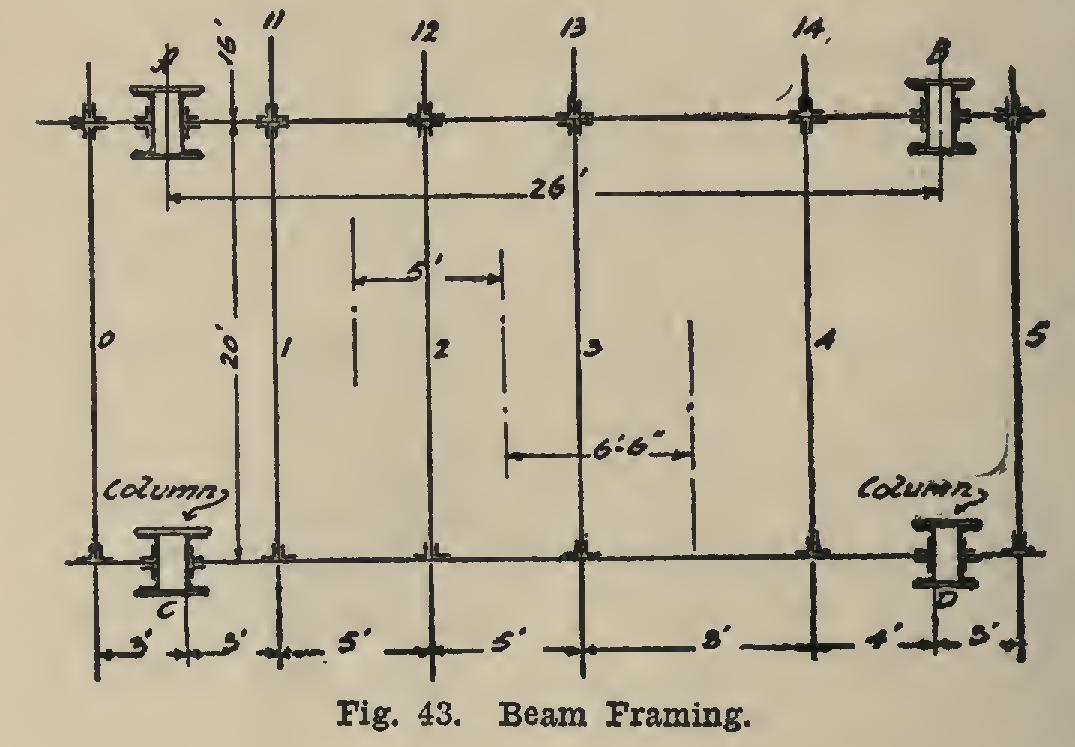Home >> Radfords-cyclopedia-of-construction-vol-4-masonry-wiring >> 325 At Load Moment to Wrought Iron 9 >> Design of Beams 38_P1

Design of Beams 38

beam, square, feet, pounds, floor, load, foot and span

Page: 1 2 3 4

DESIGN OF BEAMS 38. The design of beams is a simple matter, once the character, amount, and position of the loads that come upon them are determined.

The case of uniform loads will first be con sidered. The •usual case is where a series of beams run side by side at a certain distance apart, and these carry the floor as in Fig. 43, which is a view looking down on top of the floor. They may be spaced equal distances apart, or they may not. In the figure, the span of all the beams is 20 feet, and the first three are spaced 5 feet apart, while the last is 8 feet from the third beam.

The loads that are to come on a beam are usually stated as so many pounds per square foot of floor surface, and the weight of the floor material is given in pounds per square foot. These values are different for different cases. The weights of floors can be ascertained from any architect. Some kinds of floors are shown in C 48-49. The weights of floor per square foot will vary from 10 pounds for simple two thickness wood, to 50 pounds or more for rein forced concrete. The so-called live load, or load which may be put on the floor after it is built, will be given by the specifications. This varies from 100 pounds per square foot for ordinary office buildings, to 700 or 800 pounds or more for warehouse floors or floors on which heavy ma chinery is to be placed. All that is required, then, is to find out the number of square feet there are on which if a load be placed the entire weight will come upon the beam in question, and then multiply this by the weight of the load on a single square foot.To determine the number of square feet as above described, multiply the span of the beam by the sum of the distance to the beams on each side of it, and divide the result by 2.

For example, let it be required to determine the number of square feet on which, if loads were placed, the beam 2, Fig. 43, would be stressed.

20x (5+5) +2=100 square feet.

The computation is as follows: This may be stated another way as follows: Multiply the span of the beam by the distance from the center between beams on one side to the center between beams on the other side; or, if the beams are spaced equal distances apart, multiply the span by the distance between beams.

In case of beam 2 in Fig. 43, these last two rules give 5X20=100 square feet the same as before.

For beam 3, Fig. 43, the spacing is unequal.

The first method gives 20X (5+8)÷2=130. The second method gives 20 X 6.5=130. The third

method cannot be applied, since the spacing is not the same. Beam 1 has 110 square feet, and beam 4 has 150 square feet. The computation of this is left to the reader.

Supposing that the floor material is such that it weighs 15 pounds per square foot, and the specifications demand that the floor shall hold a load of 300 pounds per square foot, the total loads on the beams are now computed as follows: First Method of Design. Once the total load is known, and the kind of beam that is to be used, the beam may be taken from the tables in the handbook if the specifications allow a unit stress of 16,000 pounds. On pages 70 to 80 inclu sive, Carnegie Handbook, are tables giving the number of tons a beam of certain kind, size, and span will carry. A ton is 2,000 pounds, and therefore the above values must be divided by 2,000 in order to reduce them to tons. These loads are: If the beam is to be an I-beam, and beam 3 is to be designed, we must find the span 20 feet in the first column, and look across until we find a value of 20.48 or a little greater. On (C 70), under a 15-inch 60-lb., is found 21.65, and this shows that the beam we require is some place between this and the 15-inch 42-lb., which is the next column to the right and which gives 15.71 tons. By (C 97) it is seen that the I-beams be tween 42-lb. and 60-lb. are 45, 50, and 55-lb. sec tions. On pages 70-74, in the column to the right of the values of tons for any particular sized I-beam or channel, is the amount of a ton that can be added to the value given in the columns to the left in order that the tons for an I-beam or channel not given in these tables may be com puted. The number of tons which the 45, 50, and 55-pound I-beams will hold are computed as follows, the span being 20 feet in each case (C 70) : The 0.20 is obtained from the column to the right of the 15.71. Since all of these values are smaller than the 20.48 which the beam is to carry, they will not do. It is noted that these tables take into account the weight of the beam, and so this is to be deducted in order to get the actual carrying capacity. The weight of the beam is (60X20)÷2,000=0.6; and the load, in addition to its own weight, which the 15-inch 60-lb. beam can carry is (21.65-0.60)=21.05. The 15-inch 60-lb., with a carrying capacity of 21.65, including its own weight, comes the near est to the required, and could be used.

Page: 1 2 3 4